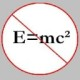You are currently browsing the tag archive for the ‘theory’ tag.

This one, by Dr. Titu Andreescu (of USAMO fame), is elementary in the sense that the solution to the problem doesn’t require anything more than arguments involving parity and congruences. I have the solution with me but I won’t post it on my blog until Jan 19, 2008, which is when the deadline for submission is. By the way, the problem (in the senior section) is from the$6^{th}$ issue of Mathematical Reflections, 2007.

Problem: Find the least odd positive integer$n$ such that for each prime$\displaystyle p, \, \frac{n^2-1}{4} + np^4 + p^8$ is divisible by at least four (distinct) primes.

I found this elementary number theory problem in the “Problem Drive” section of Invariant Magazine (Issue 16, 2005), published by the Student Mathematical Society of the University of Oxford. Below, I have included the solution, which is very elementary.

Problem: Find all ordered pairs of prime numbers$(p,q)$ such that$p^q + q^p$ is also a prime.

Solution: Let$E = p^q+q^p$. First, note that if$(p,q)$ is a solution, then so is$(q,p)$. Now,$p$ and$q$ can’t be both even or both odd, else$E$ will be even. Without loss of generality, assume$p = 2$ and$q$ some odd prime. So,$E = 2^q + q^2$. There are two cases to consider.

Case 1:$q = 3$.

This yields$E = 2^3 + 3^2 = 17$, which is prime. So,$(2,3)$ and, hence$(3,2)$ are solutions.

Case 2:$q > 3$.

There are two sub-cases to consider.$1^{\circ}:$$q = 3k+1$, where$k$ is some even integer. Then, we have$E = 2^{3k+1} + (3k+1)^2 \equiv (-1)^k(-1) + 1 \equiv -1 + 1 \equiv 0 \pmod 3$. Hence,$3 \mid E$; so,$E$ can’t be prime.$2^{\circ}:$$q = 3k+2$, where$k$ is some odd integer. Then we have$E = 2^{3k+2} + (3k+2)^2 \equiv (-1)^k(1) + 1 \equiv -1 + 1 \equiv 0 \pmod 3$. Hence,$3 \mid E$; so, again,$E$ can’t be prime.

As we have exhausted all possible cases, we conclude$(2,3)$ and$(3,2)$ are the only possible solutions.

### Blog Stats

• 346,162 hitsprof dr drd horia or… on My first postprof drd horia orasa… on My first postprof dr mircea orasa… on Inequality with lognotedscholar on Self-referential Paradoxes, In…prof dr mircea orasa… on Inequality with logprof dr mircea orasa… on Inequality with logprof dr mircea orasa… on 2010 in reviewkenji on Basic category theory, Iprof dr mircea orasa… on Solution to POW-10: Another ha…prof drd horia orasa… on Continued fraction for eprof drd horia orasa… on Inequality with logprof dr mircea orasa… on Solution to POW-12: A graph co…prof dr mircea orasa… on The Character of Physical…prof dr mircea orasa… on Milk and Tea puzzleprof drd horia orasa… on Inequality with log